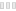# Graphing Calculator 3D

CleanPlot math equations, graphs in 2D / 3D"
A review by John

When it comes to productivity, as a student, you need an app such as this to help improve your performance. This is a handy app that allows you to plot detailed mathematical graphs to solve related equations and data problems. It is an easy-to-use application, and it allows you to make different types of graphs in a convenient workspace interface. Things you can do with this app include plotting of 2D & 3D functions, implicit equations, parametric equations, inequalities, contour graphs, create tables of values, cartesian coordinates, polar and cylindrical coordinates, spherical coordinates, import CSV & Excel coordinates, high precision computations, import live data coordinates, 3D shading & glass effects, fast rotation & graph animations, simultaneous multiple graphs, and quite a lot more.

This application also features a built-in 3D viewer so that it can present the results from your inputs in 3D. It is an advanced calculator stocked with advanced calculation tools. You can save the graphs your plot with this app as high-resolution images. Plus, you can animate graphs, as we as plot unlimited simultaneous graphs. In summary, this is a great app for every student studying mathematics in the college of high-school. It also comes in handy for advanced mathematics learners or just anyone who is interested.

Graphing Calculator 3D is licensed as freeware for PC or laptop with Windows 32 bit and 64 bit operating system. It is in mathematics category and is available to all software users as a free download.

 Share Give a rating(0 votes, average: 0.00 out of 5)login to voteLoading... Author Runiter Company Last Updated On September 15, 2019 Runs on Windows 10 / Windows 8 / Windows 7 / Windows Vista / XP Total downloads 178 License Free File size 102,45 MB Filename GraphingCalculator.zip

### Similar programsMATLAB
Tool for math calculationsMathType
Create and edit equations useful for MathML, LaTeX, TexRedCrab
Calculator for mathematical expressions
FreePrime95
Can find Mersenne Prime numbers. Stress CPU
FreeGraph
Create mathematical graphs
Free
SoftFamous
X